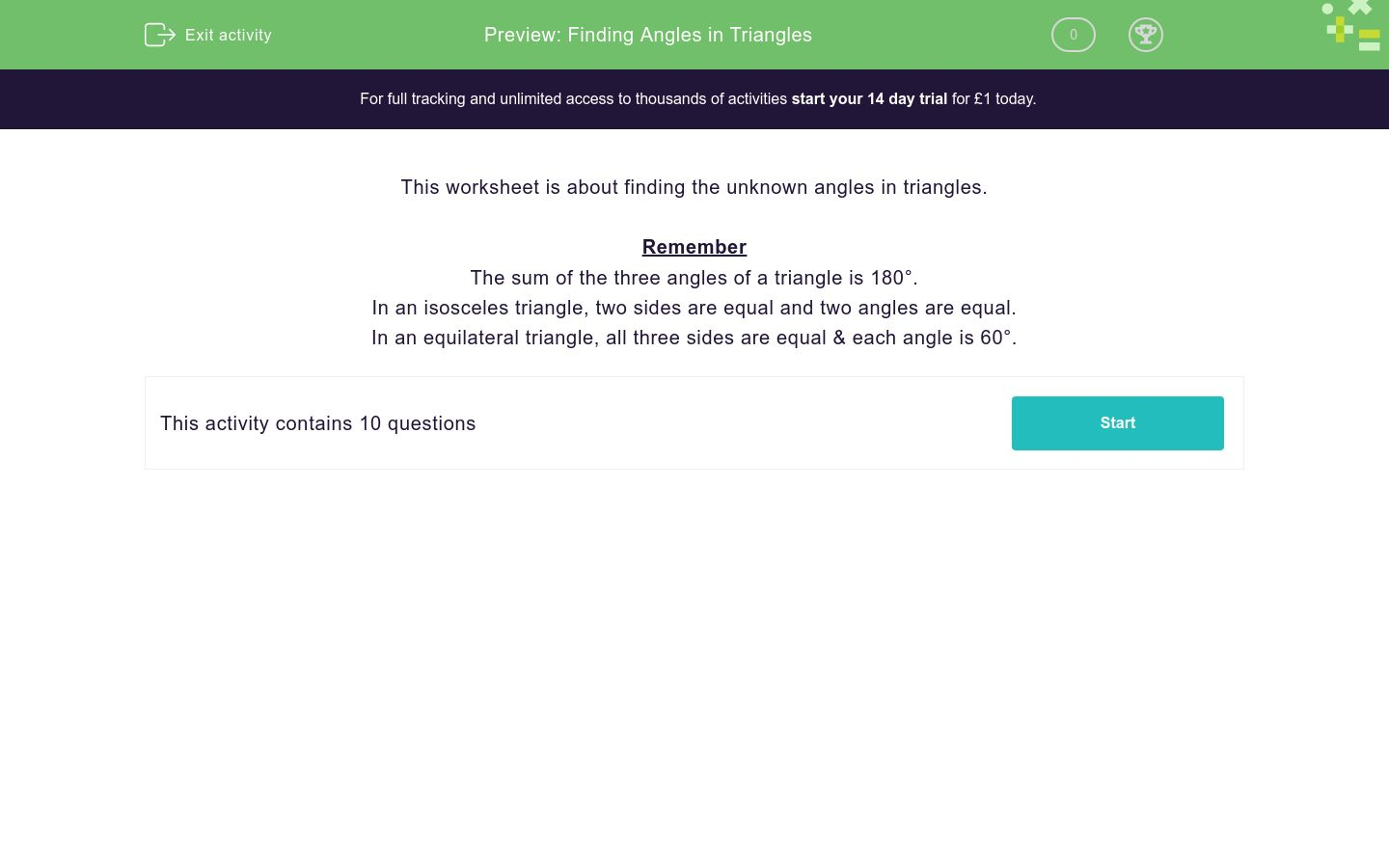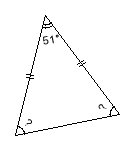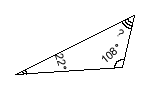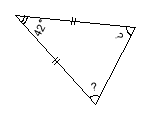# Finding Angles in Triangles

In this worksheet, students find the unknown angles in triangles.Key stage:  KS 4

Curriculum topic:  Geometry and Measures

Difficulty level:### QUESTION 1 of 10

This worksheet is about finding the unknown angles in triangles.

Remember

The sum of the three angles of a triangle is 180°.

In an isosceles triangle, two sides are equal and two angles are equal.

In an equilateral triangle, all three sides are equal & each angle is 60°.

Find the unknown angle in the triangle.(just write the number)

Find the unknown angle in the triangle.What sort of triangle is this?isosceles

equilateral

scalene

Find the unknown angle in the triangle.What sort of triangle is this?isosceles

equilateral

scalene

Find the unknown angle in the triangle.Find the unknown angle in the triangle.Find the unknown angle in the triangle.Find the unknown angle in the triangle.Find the unknown angle in the triangle.• Question 1

Find the unknown angle in the triangle.(just write the number)

CORRECT ANSWER
47
EDDIE SAYS
180 - 104 - 29 = 47
• Question 2

Find the unknown angle in the triangle.CORRECT ANSWER
29
EDDIE SAYS
180 - 104 - 47 = 29
• Question 3

What sort of triangle is this?CORRECT ANSWER
isosceles
EDDIE SAYS
Isosceles triangles have two equal sides.
• Question 4

Find the unknown angle in the triangle.CORRECT ANSWER
45
EDDIE SAYS
180 - 90 (right angle) - 45 = 45
Again, this is an isosceles triangle - you can tell by the two short double lines on the sides of the triangle that are the same length.
• Question 5

What sort of triangle is this?CORRECT ANSWER
equilateral
EDDIE SAYS
Equilateral triangles have three equal sides, meaning that all three angles are also the same (60°).
• Question 6

Find the unknown angle in the triangle.CORRECT ANSWER
50
EDDIE SAYS
180 - 108 - 22 = 50
• Question 7

Find the unknown angle in the triangle.CORRECT ANSWER
63
EDDIE SAYS
180 - 90 - 27 = 63
• Question 8

Find the unknown angle in the triangle.CORRECT ANSWER
69
EDDIE SAYS
(180 - 42) ÷ 2 = 69
• Question 9

Find the unknown angle in the triangle.CORRECT ANSWER
60
EDDIE SAYS
180 ÷ 3 = 60
Again, this is an equilateral triangle, so all of the angles will be 60°.
• Question 10

Find the unknown angle in the triangle.CORRECT ANSWER
64.5
EDDIE SAYS
(180 - 51) ÷ 2 = 64.5
---- OR ----

Sign up for a £1 trial so you can track and measure your child's progress on this activity.

### What is EdPlace?

We're your National Curriculum aligned online education content provider helping each child succeed in English, maths and science from year 1 to GCSE. With an EdPlace account you’ll be able to track and measure progress, helping each child achieve their best. We build confidence and attainment by personalising each child’s learning at a level that suits them.

Get started#### Similar activities you may like

Start your £1 trial today.
Subscribe from £10/month.